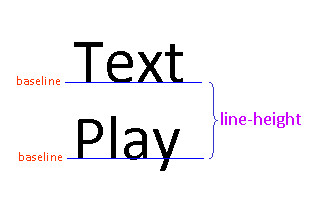# CSS Line-Height Property

The line-height property specifies the amount of space between the baselines of two lines. A baseline is the bottom of most letters. The figure below illustrates what line-height represents:Note that line-height is not the amount of space between two lines.

Possible values for line-height include the following:

• A number: the amount of space between baselines is equal to this number multiplied by the font size. Negative numbers are ignored, while decimals are allowed. While it is permissible to set a number that is very small (close to zero), doing so would cause the lines to overlap each other and hence is usually not a good idea.
• A percentage: the amount of space between baselines is equal to this percentage multiplied by the font size.
• A length: this is the absolute length between baselines. Unlike the other two types of values, it is not dependent on font size.

We show several examples below.

Example 1: Using a number for line-height

CSS Declaration

 p {   line-height: 2; }

The following HTML code,



This paragraph illustrates setting the amount of space between two lines using a number.

renders

This paragraph illustrates setting the amount of space between two lines using a number.

In Example 1, we set line-height to 2, which is equivalent to using double space between lines.

Example 2: Using a percentage for line-height

CSS Declaration

 p {   line-height: 80%; }

The following HTML code,



This paragraph illustrates setting the amount of space between two lines using a percentage.

renders

This paragraph illustrates setting the amount of space between two lines using a percentage.

In Example 2, we set line-height to 80%, and that causes the lines to appear to be squeezed together.

Example 3: Using a length for line-height

CSS Declaration

 p {   line-height: 45px; }

The following HTML code,



This paragraph illustrates setting the amount of space between two lines using a length.

renders

This paragraph illustrates setting the amount of space between two lines using a length.

In Example 3, we set line-height to 45px. When using an absolute length for line-height, be extra cautious as you need to make sure the amount of space between lines is compatible with the font size.

Next: CSS Text-Align